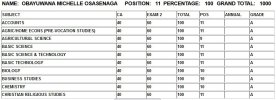# Positioning student result by ranking grade

#### agocomp

I've created a ranking system for result that contains student score based on subject from 1 to 100 percent, the application worked but when a student gets 100 percent the system ranks the student last in class. The expectation is that the system should rank the student with 100 percent as 1st in class.
Below is the code:

Code:
``````<?php
session_start();
include("DB/config.php");
ini_set('max_execution_time', 1000000);

\$class_arm= \$_SESSION['clas'];

\$queris = "select * from setup";
\$result3 = mysqli_query(\$con,\$queris) or die(mysqli_error(\$con));
\$rws3 = mysqli_fetch_assoc(\$result3);
\$ses3=\$rws3['section'];
\$term3=\$rws3['term'];

\$query_sj = "SELECT subj FROM sec_result where class='\$class_arm' and year='\$ses3' and term='\$term3' and tsc<>'' and tsc is not null and tsc <> '0'";
\$result_sj = mysqli_query(\$con,\$query_sj) or die(mysqli_error(\$con));

while(\$row_sj = mysqli_fetch_array(\$result_sj)){

\$query = "SELECT distinct studid,subj,tsc FROM sec_result where class='\$class_arm' and year='\$ses3' and term='\$term3' and subj='".\$row_sj['subj']."' ORDER BY tsc DESC";
\$result = mysqli_query(\$con,\$query) or die(mysqli_error(\$con));

/*\$row2 = mysqli_fetch_array( \$result );
echo \$row2['tsc'];
exit;*/

if( !\$result ){

echo 'SQL Query Failed';

}else{

\$rank = 0;

\$last_score = false;

\$rows = 0;

while( \$row = mysqli_fetch_array( \$result ) ){

\$rows++;

if( \$last_score!= \$row['tsc'] ){

\$last_score = \$row['tsc'];
\$nuval = \$row['tsc'];

\$rank = \$rows;

}

mysqli_query(\$con,"UPDATE sec_result SET pos = '\$rank' where studid='".\$row['studid']."' and year='\$ses3' and term='\$term3' and subj='".\$row_sj['subj']."'") or die(mysqli_error(\$con));

//echo "rank ".\$rank." is ".\$row['studid']." wit

//h point ".\$row['avg'] . "\n";

}

}
}
?>``````

#### Attachments

•result.jpg
109.7 KB · Views: 3

#### thatstooeasyman

The issue with the code is that the ranking is based on the student's score, and the ranking is assigned based on the previous student's score. So, when a student gets 100%, the ranking assigned to the student is based on the previous student's score, which is less than 100%. To fix this issue, you can modify the code to check if the current student's score is 100% and assign the rank accordingly.

Here's the modified code:

PHP:
``````<?php
session_start();
include("DB/config.php");
ini_set('max_execution_time', 1000000);

\$class_arm= \$_SESSION['clas'];

\$queris = "select * from setup";
\$result3 = mysqli_query(\$con,\$queris) or die(mysqli_error(\$con));
\$rws3 = mysqli_fetch_assoc(\$result3);
\$ses3=\$rws3['section'];
\$term3=\$rws3['term'];

\$query_sj = "SELECT subj FROM sec_result where class='\$class_arm' and year='\$ses3' and term='\$term3' and tsc<>'' and tsc is not null and tsc <> '0'";
\$result_sj = mysqli_query(\$con,\$query_sj) or die(mysqli_error(\$con));

while(\$row_sj = mysqli_fetch_array(\$result_sj)){

\$query = "SELECT distinct studid,subj,tsc FROM sec_result where class='\$class_arm' and year='\$ses3' and term='\$term3' and subj='".\$row_sj['subj']."' ORDER BY tsc DESC";
\$result = mysqli_query(\$con,\$query) or die(mysqli_error(\$con));

if(!\$result){
echo 'SQL Query Failed';
}else{

\$rank = 0;
\$last_score = false;
\$rows = 0;

while(\$row = mysqli_fetch_array(\$result)){

\$rows++;

if(\$last_score!= \$row['tsc']){
\$last_score = \$row['tsc'];
\$nuval = \$row['tsc'];
\$rank = \$rows;
}

//check if the current student's score is 100% and assign the rank accordingly
if(\$row['tsc']==100){
\$rank = 1;
}

mysqli_query(\$con,"UPDATE sec_result SET pos = '\$rank' where studid='".\$row['studid']."' and year='\$ses3' and term='\$term3' and subj='".\$row_sj['subj']."'") or die(mysqli_error(\$con));

//echo "rank ".\$rank." is ".\$row['studid']." with point ".\$row['avg'] . "\n";
}
}
}
?>``````